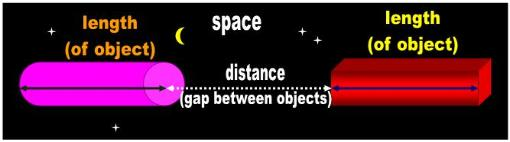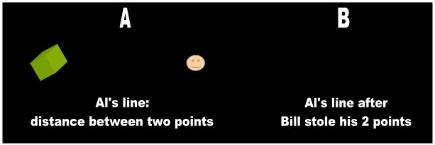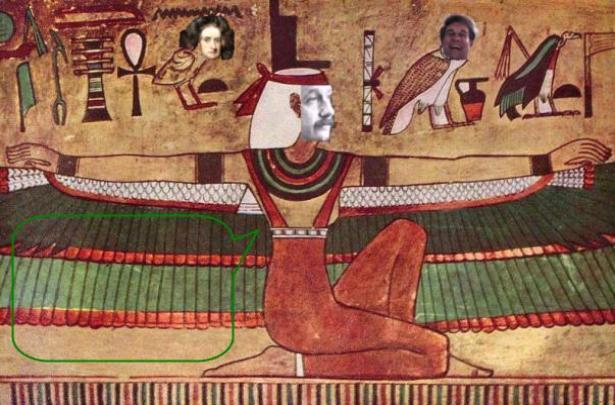1.0    Is a line equal to two points or to the distance between them?

The most common definition of a straight line is the ever popular 'shortest distance':

To draw a straight-line from any point to any point.”
(Book I, Postulate 1)  

[Meaning…]

Any two points can be joined by a straight line.”  

A geodesic is the shortest (or longest) path between two nearby points...In
general relativity, bodies always follow straight lines (p. 29) 

Two-points and the distance between them are obviously not equivalent (Fig. 1). Either a line consists of two points or
a line is what lies between two points. It can't be both. The ‘shortest distance’ assumes the latter. Therefore, if a line is
what we draw or can draw after we set up our two points, the two points all by themselves do not constitute a line, a
straight line, or a line segment. This objection summarily debunks the two-point definition and shows that the
proposals of Mathematics are not as rigorous as advertised.

Obviously, before Bill removes Al's points, the mathematicians expect him to fill the void between the dots with something:

“The distance between two points in space can be ascertained with a rod”  

Can be? So what have we learned? What is the line now? Is the line a trace that we will be drawing with our pencil, the rod
which we place between the empty locations, or the empty space between two dots?

4.0   Distance between concepts?

The mathematical physicists ask you to believe that a point is really a location. The dot is merely a pictorial representation
of this location:

“ Objects in 1D are bounded by 0D points” 

A point shows an exact position or location...a point is not a thing, but a
place... We draw a point by placing a dot 

Pursuant to these definitions, the famous line ends up being a void between two voids. We have two locations with
nothing occupying them and an abstract distance between them (Fig. 1 B). Again, the mathematicians are expecting the
audience to fill in the blanks. Without a physical marker, there is no such concept as location. If relativists artificially place
two dots to mark the respective locations, then we are not talking abstract locations, but about physical dots. The
mathematicians use the dot to mark and then talk about abstract locations throughout the rest of the presentation.

Just in case, locations are not the only abstract concepts between which the mathematicians claim they can measure
distances. They also claim to measure distances between numbers, sets, ordered pairs, and events:

“ the usual definition of distance between two real numbers x and y is... the distance
between two different but overlapping sets will be found to be equal”

Calculate the distance from A to B in each of the following: a) A (3,5); B(6,9),
b) A (-2,4): B(3, -8) ... 

“ the distance between any two events in spacetime (called an interval) may be real,
zero, or imaginary.”  

The distance between two events? The distance between two numbers?

So what’s the distance between anger and happiness, two events that happened to me yesterday at around 2:00 o’clock?
How about between the numbers 5 and 7? Where are these numbers located?

You wonder what it is that the mathematicians really drink when they shoot the bull at the ‘water cooler.’ After dealing
with abstractions for so long, the mathematicians have lost complete track of what they were talking about.

5.0   Is the shortest distance a geometric figure?

Assuming we allow the mathematician to proceed with the 'shortest distance between,' one wonders whether the result
will be a valid geometric figure that can be used in the exhibits phase of a scientific hypothesis. Is the side of a cube an
edge or a distance? The word line alludes  to a ‘something’ whereas a distance refers to a ‘nothing.’ In Physics, a distance
is a synonym of void: the absence of things between two static points. Distance is a word we use to refer to the gap or
separation between TWO objects. There must absolutely be two surfaces present to understand the concept distance. A
length is exactly the opposite. A length refers to the qualitative extent of ONE object. We could synthesize the two notions
in a neat little statement that mathematicians should learn to memorize in Kindergarten Geometry 101:

distance is what lies between lengths (Fig 2).

If a line is what has length, a distance is what doesn't. The infamous 'distance' of Mathematical Physics is not a part of
Geometry!

And now for the punch line... After so much talk, the mathematician throws in a disclaimer. It turns out that the 'distance' that
the mathematician was talking about for the last two hours is not the distance of Physics. The mathematician was referring
to the 'distance-traveled' by an object. That's Hawking's geodesic version of a line.
 Fig. 2   Homework: Memorize the following axiom of Physics by heart"distance is what lies between lengths"“ the straight line lies evenly between its points” 

However, there are more serious conceptual problems with the ‘shortest distance’ definition of Mathematics:

it is an incongruous attempt to prove a definition
the mathematicians give lip service to the between provision and routinely use beyond
it is irrational to talk about a distance between two abstract concepts
it equates distance (actually distance-traveled) with the geometric figure line
it assumes that distance is continuous

2.0   Proof

In Physics, the definition of a structure should not embody a challenge. The word ‘shortest’ dares the skeptic to verify that
this is indeed the case. This means that there must be another distance or line to compare the present one against.
Consequently, the definition of a line involves two lines and for the proponent to be there to relate them (i.e., to prove that
one is shorter or longer than the other). Meanwhile, the ET must wait to understand what a line is until the moron of relativity
finds a second line somewhere in the woods. Just in case, it would be unwise for relativists to insist that a geodesic is by
definition the shortest of all distances. They might lose more than their pride.

Nevertheless, in Science, and particularly in Physics, the design of the experiment precedes the experiment. Before you
run a test or make a deliberate observation you must conceptualize, you must visualize the entire movie in your head.
What are you expecting to prove or disprove? What do you hope to achieve? And before you can visualize or
conceptualize you must define the key words and identify the key objects that play a relevant role in your theory. In
Science, we must know what the word line means before speaking about it, proving something, or doing anything with it.

The mathematicians never follow this sequence. They would rather do science exactly in reverse. The mathematicians
run a test to conceptualize or visualize what they were doing for the last two hours, and end up proving a definition. For
example, the folks at places like LIGO and Gravity Probe B (GPB) have yet to define the key words that make or break the
theories they are attempting to confirm. The mathema-ticians at GPB are attempting to prove that space is a warped
physical object and that the Earth rubs its belly against this entity. As a minimum, we need unambiguous definitions of the
words space, object, warped, and touch (i.e., drag) before we discuss the matter. The most important within this set -- space
and object -- have never been defined in a way that we can use them consistently (i.e., scientifically). So what are the bozos
at NASA and Stanford testing? What is it that they hope to achieve? How can they be at the experimental stage if they have
not conceptualized what they are hoping to achieve or even defined the crucial words that make or break their experiment?

Let's now do it the scientific way. We begin with definitions. We define the word  space as 'that which has no shape' and the
word object as 'that which has shape.' Pursuant to these definitions, it is irrational to propose that space is warped or that it
can be dragged around by an object such as a planet. Until we resolve this conceptual inconsistency there is no justification
to run any tests, meaning that the bozos at NASA wasted \$600 million in tax dollars.

The mathematicians apply the same irrational methodology of GPB when they ask an ET to infer the 'shortest distance' line
of Mathematical Physics. The scholars do not tell this visitor what a line is nor do they define it for him. What they do is
assign homework. The ET is supposed to go to the store and buy two points and a pencil. He places the two points far
apart, puts the tip of the pencil on the first one, and begins to drag the pencil as he walks towards the other one. They tell
the ET that upon completion of the experiment he will know what a line is. If the ET places the two points one mile apart,
he won't know what a line is for another half an hour. If he takes the second point to the other side of the Universe (i.e., the
infinite line), he may never know what the mathematicians were referring to.

In Science we don’t prove or define geometric figures such as lines. In Science, we point to them and utter a sound: "line."
We're done!

The mathematician may remark that this version of 'line' is not very useful.

However, Science is unconcerned with whether a mathematician or a philosopher can use the object we just pointed to.
This is WHAT a line IS! If the mathematician can't use it, he should find something he can and refrain from calling it a line.

3.0   The Infinite line: between vs. beyond

The mathematicians claim that the authentic straight line extends indefinitely in two directions.

“ Given any two distinct points in space there is a unique line that passes through
both of them.”  

“ Given any two points, there exists a line containing both of them.” (Axiom I.1)...there
exists a point B on the line AC such that C lies between A and B. (Axiom II.2) 

[Since Hilbert and his successors weren't smart enough to see what is wrong with
these two definitions, I guess I'll have to spell it out. The first definition deals with a
line (i.e., infinite). The second set of definitions refers to a line segment (i.e., finite).
Hilbert is comparing apples and oranges. His idiotic 'axioms' are worthless.]

The entity capped by endpoints is technically a line segment:

“ line segment: A portion of a line that has a beginning and an end. A line segment
can be measured.”   

These irreconcilable definitions and the use of the word line to designate both the infinite as well as the finite line are
guaranteed to generate confusion. Either a straight line is what exists between two points or includes in addition what
extends beyond it. Relativists, so fond of dualities, want it both ways, and this explains why they created the irrelevant
concept known as the line segment:

“ Lines are used to construct all other figures in plane geometry, including angles,
triangles, squares, trapezoids, circles, and so on. Since a line has no beginning
or end, most of the ‘lines’ one deals with in geometry are actually line segments
— portions of a line that do have a limited length.” 

The infamous line segment is irrelevant because no one on planet Earth ever used or uses it. In Mathematical Physics,
everybody and his mother gives lip service to the line segment and calls it a line. During the dissertation, the 'line-segment-
turned-line' magically outgrows its endpoints as if they weren't there. The reason for all of this is simple: a mathematician
has no use for the genuine line of Geometry. The line segment is one of those concepts that every mathematician on Earth
rapes and pummels and treats like a slut! Then he dumps her for the line. If the true-blue, unadulterated, relativistic line will
extend beyond the stop signs, what was the purpose of the two points? What is the purpose of a line segment if the
mathematicians are always going to go beyond the points to prove their theorems?

The problems with Euclid's ‘infinite distance’ line don't stop there. A more serious shortcoming is that  it is still under
construction. His infinite line is a promise, a ne’er-to-be-finished sculpture. And if he ever manages to finish it, this ever
growing, dynamic line is guaranteed not to be rectilinear let alone straight. It is irrational to say that a movie of an object in
motion is 'straight.'

On the other hand, if the ‘distance between’ refers to the void between two points, the definition of straight line cannot do
without the two physical endpoints. It is irrational to attempt to establish a distance between two concepts, say, between
love and hate or between justice and beauty. We absolutely require two surfaces to talk about between in Physics.

But then, a simple test ought to show whether this 'distance' line qualifies as a geometric figure. Bill removes the two dots
(Fig. 1). Is this what a straight line is? The empty space that remains behind?
 Fig. 1The mathematical line: distance between 2 points
 If the mathematician says that a line is the distance between two points, we can easily determine whether this definition can be used in Geometry. We remove the two irrelevant points. Is what remains behind a line?You see, Bill! The line is the distance between my hands. This is the longest distance possible in Geometry because I can't stretch them any further.So far, all you have is a point, Bill. Now drag it across like this. That's what we call a line
 Adapted for the Internet from:Why God Doesn't Exist
 Weyl's shortest distance________________________________________________________________________________________